## IRIS dataset¶

#### Exercise 1.1¶

To load the dataset, the csv module can be used. This allows iterating over the entire dataset.

Based on the following exercises, it makes sense to store the CSV file as a list of 5 columns. These will be stored in the dataset variable.

Notice how we are making sure that the row list always has 5 elements before processing it. It just so happens that the last row of the iris.csv file contains an empty line, thus making this check necessary.

In :
import csv

dataset = [ [], [], [], [], [] ]
measurements = [ "sepal length", "sepal width", "petal length", "petal width" ]
with open("iris.csv") as f:
if len(row) == 5: # only do this if the number of columns is 5, as expected
for i in range(4): # the 4 measurements should be converted to float
dataset[i].append(float(row[i]))
# position 4 is the iris type, which is to be kept as a string
dataset.append(row)


#### Exercise 1.2¶

Now that each of the elements of dataset contain one of the columns of the Iris dataset, the second exercise consists in computing the mean and the standard deviation of the first 4 elements (lists) of dataset.

First, we need to define a couple of functions that compute the mean and the standard deviation of a list of values.

Let's begin with the mean.

In :
def mean(x):
return sum(x) / len(x)


The sum() function comes in handy. It sums all the values of a given list. We then divide this sum by the length of the list x, thus effectively computing the mean value.

The standard deviation, on the other hand, can be seen as the square root of the mean squared difference of each element of x from the mean, squared.

Let's break this down with this toy example:

In :
x = [ 5, 3, 15, 4, 1 ]
u = mean(x)
u

Out:
5.6

The squared difference of each element from the mean u (which we have computed using the previously defined mean() function) is given by:

In :
diff = [ (x_i - u) ** 2 for x_i in x ]
diff

Out:
[0.3599999999999996,
6.759999999999998,
88.36000000000001,
2.5599999999999987,
21.159999999999997]

Once we have computed this difference, we can compute its mean. The mean() function we have already created comes in handy. Then, we compute its square root.

In :
print(mean(diff)**0.5)

4.882622246293481


By putting it all together, we get this function.

In :
def std(x):
u = mean(x)
return (mean([ (x_i - u) ** 2 for x_i in x ])) ** 0.5


Let's make sure it works as expected with our toy example.

In :
std(x)

Out:
4.882622246293481

Now, we can apply these two functions to our columns.

In :
for i, m in enumerate(measurements):
print(f"{m} mean {mean(dataset[i]):.4f} std {std(dataset[i]):.4f}")

sepal length mean 5.8433 std 0.8253
sepal width mean 3.0540 std 0.4321
petal length mean 3.7587 std 1.7585
petal width mean 1.1987 std 0.7606


#### Exercise 1.3¶

We now need to do what we did in exercise 1.2, but for each Iris type separetely.

First, let's identify the various Iris types available. We may be tempted to create a list of values such as the following:

In :
iris_types = [ 'Iris-setosa', 'Iris-versicolor', 'Iris-virginica' ]


With this approach, though, we are going to need to update the code every time a new Iris type is added. Additionally, this solution might work well with 3 Iris types, but we will probably not do this for a problem that has 300 types. Instead, since we already have a list of all possible types in dataset, we can remove all duplicates by creating a set out of it.

In :
iris_types = set(dataset)
iris_types

Out:
{'Iris-setosa', 'Iris-versicolor', 'Iris-virginica'}

With this out of the way, we can now iterate over each column and, for each column, over each Iris type. Then, we select all values for that measurement and that Iris type and compute mean and standard deviation.

In :
for i, m in enumerate(measurements):
print(m)
for iris_type in iris_types:
# For each measurement and for each iris type, build a list of values
values = [ v for v,t in zip(dataset[i], dataset) if t == iris_type ]
print(f"{iris_type} {mean(values):.4f} {std(values):.4f}")
print()

sepal length
Iris-virginica 6.5880 0.6295
Iris-setosa 5.0060 0.3489
Iris-versicolor 5.9360 0.5110

sepal width
Iris-virginica 2.9740 0.3193
Iris-setosa 3.4180 0.3772
Iris-versicolor 2.7700 0.3106

petal length
Iris-virginica 5.5520 0.5463
Iris-setosa 1.4640 0.1718
Iris-versicolor 4.2600 0.4652

petal width
Iris-virginica 2.0260 0.2719
Iris-setosa 0.2440 0.1061
Iris-versicolor 1.3260 0.1958



#### Exercise 1.4¶

Based on the previous results, the measurement that most helps discriminate among the three Iris types is the one that has the most distant means and the smallest standard deviations.

This corresponds to distributions of values that are well separated (distant means) and all fall within a short distance on the mean value (small standard deviation).

At a first look at the previous values, the one that jumps out is the petal width. The means are well separated (approximately 0.2, 1.3 and 2.0) and the standard deviations are low (all below 0.27).

A visual aid might help with this kind of considerations. Although not yet introduced, the following code will plot a bell curve and the actual histograms for each of the three Iris types, for each of the measurements. The means and standard deviations of the curves are the one previously computed.

In :
import matplotlib.pyplot as plt
%matplotlib inline
from scipy.stats import norm
import numpy as np

colors = ['b','g','r']
for i, m in enumerate(measurements):
plt.figure()
plt.title(m)
for iris_type, color in zip(iris_types, colors):
# For each measurement and for each type of iris, build a list of values
values = [ v for v,t in zip(dataset[i], dataset) if t == iris_type ]
plt.hist(values, density=True, alpha=0.2, color=color)
u = mean(values)
s = std(values)
x = np.linspace(u-5*s, u+5*s, 100)
plt.plot(x, norm(u,s).pdf(x), label=iris_type, color=color)
plt.xlabel(f"{m} (cm)")
plt.ylabel("density")
plt.legend()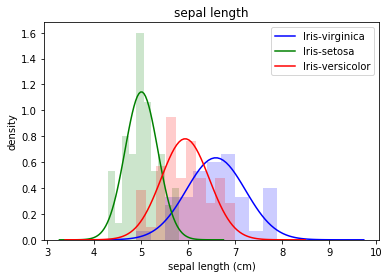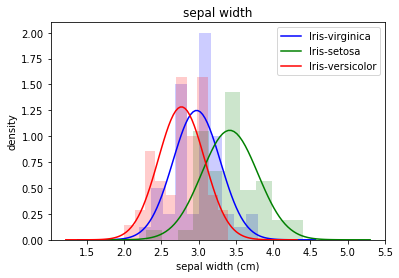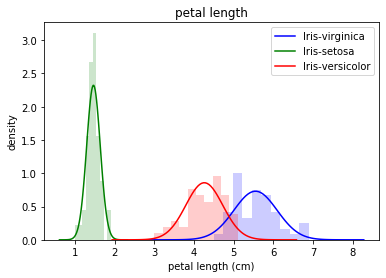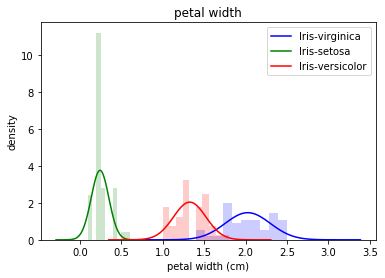As expected, the the bell curves for the petal width are fairly well separated. The same happens for the petal length (another good separator for the three classes). Instead, sepal length and width are worse discriminators (i.e. they are not particularly helpful in separating the three classes).

Finally, notice how much different Setosa is from Virginica and Versicolor. This makes telling whether a flower is an Iris Setosa an easier task, compared to Versicolor or Virginica.

## Citybik.es dataset¶

#### Exercise 2.1¶

For this exercise, we need to rely on the json module to load a dictionary from a JSON file to the program's memory.

In :
import json
with open("bikes.json") as f:


The dataset dictionary now contains all the information needed. Since we need to work on the bike station for the following exercise, we can now explore this data structure to find where the stations are stored. We can get a list of keys for dataset by using the .keys() method.

In :
dataset.keys()

Out:
dict_keys(['network'])

Only one key, named network, is available. To understand the type of contents of dataset["network"], we can rely on the type() function.

In :
type(dataset["network"])

Out:
dict

Another dictionary. Meaning that we can, once again, access its keys.

In :
dataset["network"].keys()

Out:
dict_keys(['company', 'href', 'id', 'location', 'name', 'source', 'stations'])

Now, this is more interesting. Of the many keys availabe, one, stations, is likely to be the one we are looking for.

In :
type(dataset["network"]["stations"])

Out:
list

Now, this is a list. This makes sense, since we expect there to be a list of all stations available. Let's have a look at what one of these stations looks like.

In :
dataset["network"]["stations"]

Out:
{'empty_slots': 4,
'extra': {'number': 1,
'reviews': 1212,
'score': 3.4,
'status': 'online',
'uid': '250'},
'free_bikes': 0,
'id': '11c6ccc569e1b91fe1e9d238f97f4a32',
'latitude': 45.077589,
'longitude': 7.670385,
'name': 'Paravia',
'timestamp': '2019-09-20T12:16:42.631000Z'}

This contains all the relevant information we need for the next exercises.

#### Exercise 2.2¶

The number of active stations can be computed by only keeping the "online" stations and discarding the others. Based on the previous output, we know that the "online" value is stored in the "extra" dictionary within each station, at the key "status".

We can use a list comprehension to extract the active stations, and print their number. Notice that, in this solutions, list comprehensions are being used to perform the same task that the filter() function does.

In :
active_stations = [ station for station in dataset["network"]["stations"] if station["extra"]["status"] == "online" ]
print("Number of active stations", len(active_stations))

Number of active stations 156


#### Exercise 2.3¶

In this exercise, we need to count the total number of bikes available and the total number of free docks. This information is available at a "single station" level, so we are going to aggregate the information at a "whole system" level.

In :
bikes_avail = sum([ station["free_bikes"] for station in dataset["network"]["stations"]])
free_docks = sum([ station["empty_slots"] for station in dataset["network"]["stations"]])
print("Bikes available", bikes_avail)
print("Free docks", free_docks)

Bikes available 233
Free docks 1158


We can once again use list comprehensions. In this case, though, we are not using any filters (as was the case with the previous exercise), but rather we are extracting a value from the data structure. This is what the map() function does and, indeed, this exercise could be rewritten by leveraging that function.

In general, basic list comprehensions can be rewritten as a combination of filter() and map(). Using the one or the other might at times improve code readability, but the decision generally boils down to personal preferences.

#### Exercise 2.4¶

For this exercise, we are going to compute a distance among points, as defined by their coordinates (in terms of latitude and longitude). Since latitudes and longitudes are angles, using the Euclidean distance with those coordinates would not yield meaningful results.

The proposed approach to computing distances is based on the great-circle distance, which approximates the Earth to a sphere, and computing the distances therein (on a sphere, the shortest path between two points is the arc of a circumference centered in the same center as the sphere, and passing by the two points).

For simplicity, an implementation of the distance function has already been provided.

In :
from math import cos, acos, sin

def distance_coords(lat1, lng1, lat2, lng2):
"""Compute the distance among two points."""
deg2rad = lambda x: x * 3.141592 / 180
lat1, lng1, lat2, lng2 = map(deg2rad, [ lat1, lng1, lat2, lng2 ])
R = 6378100 # Radius of the Earth, in meters
return R * acos(sin(lat1) * sin(lat2) + cos(lat1) * cos(lat2) * cos(lng1 - lng2))


With this function, computing the distance between any two points, say (45.074512, 7.694419) and (45.075309, 7.695369) can be done as follows:

In :
# distance, in meters
distance_coords(45.074512, 7.694419, 45.075309, 7.695369)

Out:
115.96832619435945

Now, we can iterate over all stations and select the closest one to our point of interest (making sure that the station has bikes available, as request by the exercise).

We will store the information (closest_station, closest_distance) in the closest tuple. We will initialize this tuple to (None, None) (the None value is used to represent the conecpt on "nothing" (much like null in Java)).

In :
def distance_from_point(dataset, lat, lng):
closest = (None, None)
for station in dataset["network"]["stations"]:
closest_station, closest_distance = closest
current_distance = distance_coords(lat, lng, station["latitude"], station["longitude"])
# if closest_distance is None, then we are at the first
# loop execution where the station has available bikes.
# In that case, we save the current station as the
# closest one (as we do not have any other stations available).
# From the next cycle on, to update closest, we need
# the station to actually be closer than the already saved one.
if station["free_bikes"] > 0 and (closest_distance is None or current_distance < closest_distance):
closest = (station, current_distance)
return closest

station, distance = distance_from_point(dataset, 45.074512, 7.694419)
print("Closest station:", station["name"])
print("Distance:", distance, "meters")
print("Number of available bikes:", station["free_bikes"])

Closest station: Regina Margherita 3
Distance: 164.17479398512074 meters
Number of available bikes: 7


This solution, as efficient as it might be (it only iterates over the data once), is particularly verbose and kind of "reinvents the wheel": we are implementing a function that computes the minimum of a list of values, with all the problems that may come with it.

It is seldom a good idea to re-implement functions that are already available. In this case, python offers the min() and max() functions. These functions iterate over a list and identify the element having lowest/highest value.

In :
v = [ 1, -2, 3, -4, 5 ]
min(v), max(v)

Out:
(-4, 5)

This works just fine for numerical examples, where comparisons can be made easily (it makes sense to say 1 < 2, 15 < 20, 23 > 19).

In this exercise, though, our list contains stations. Saying that a station is smaller or larger than another does not make sense, if we do not specify how the comparison among the two should happen. This is where the key parameter of the min/max functions comes into play.

This key parameter is a function, called "key extractor", that can be used to extract a value from a complex object (e.g. a "station"). The min()/max() functions then use this function to extract the correct value and use it for comparison.

As an example, imagine we wanted to identify the station with the highest number of available bikes. We could use the max() function on the list of stations, specifying that, for each station, the value we want (our "key") is the field "free_bikes".

In :
max(dataset["network"]["stations"], key=lambda station: station["free_bikes"])

Out:
{'empty_slots': 7,
'extra': {'number': 40,
'reviews': 358,
'score': 4.0,
'status': 'online',
'uid': '289'},
'free_bikes': 7,
'id': '4857953654e96658febd72bc5af8bc5d',
'latitude': 45.044426,
'longitude': 7.664214,
'name': 'Giordano Bruno',
'timestamp': '2019-09-20T12:16:42.631000Z'}

Now that we know how to identify the minium value in a list, we need the distance between each station and the point of interest. We can map each station to a tuple (station, distance). We can then apply the min() function to this list of tuples.

In :
def distance_from_point_2(dataset, lat, lng):
v = [ (s, distance_coords(lat, lng, s["latitude"], s["longitude"])) for s in dataset["network"]["stations"] if s["free_bikes"] > 0 ]
return min(v, key=lambda w: w)

station, distance = distance_from_point_2(dataset, 45.074512, 7.694419)
print("Closest station:", station["name"])
print("Distance:", distance, "meters")
print("Number of available bikes:", station["free_bikes"])

Closest station: Regina Margherita 3
Distance: 164.17479398512074 meters
Number of available bikes: 7


## MNIST dataset¶

#### Exercise 3.1¶

After downloading the dataset, we can read it using the csv module, as already shown in the first exercise. In this case, though, we are interested in the rows (each one represents a digit). As such, we will be storing the CSV file by rows.

In :
import csv

dataset = []
labels = []
with open("mnist.csv") as f:
labels.append(int(cols.pop(0)))
dataset.append(list(map(int, cols)))


Now, dataset contains 10,000 lists of values, each one representing a digit (as a 784-dimensional vector). labels is instead a list of numbers, each entry is the digit represented in the respective entry of dataset.

#### Exercise 3.2¶

For any given digit, we want to print it as a 28x28 grid. Before diving right into the grid representation, we should establish a useful building block.

In particular, we will need to map any pixel value between 0 and 255 to the character we want to display. We will create a function, get_char(), with exactly this purpose.

In :
def get_char(pixel):
ranges = {
(0, 64): " ",
(64, 128): ".",
(128, 192): "*",
(192, 256): "#"
}
for (a,b),ch in ranges.items():
if a <= pixel < b:
return ch

[ get_char(0), get_char(72), get_char(192), get_char(138), get_char(250) ]

Out:
[' ', '.', '#', '*', '#']

Once we have this function, we can map any 784-dimensional vector to the corresponding sequence of characters.

With this, we can use two nested loops (one over the rows, the other over the columns) to print the resulting grid.

Note that, if we want to print the character at the i-th row and j-th column, we will need to access the (28*i+j)-th value of the 784-dimensional vector.

In :
def print_digit(dataset, digit):
chars = list(map(get_char, dataset[digit]))
for i in range(28): # iterate over rows
for j in range(28): # iterate over columns
print(chars[i*28+j], end="")
print()

In :
print_digit(dataset, 129)



.#      **
.##..*#####
#########*.
#####***.
##*
*##
##
.##
###*
.#####.
*###*
*###*
###
.##
###
.###
.    *###.
.# .*###*
.######.
*##*.



Pay close attention to the way we use print() when printing each character. We specify the end parameter as having an empty string value (""). This is the character that print appends after each call. By default, this value is a newline ("\n"). If we do not change it to an empty value, each subsequent print call would create a new line.

#### Exercise 3.3¶

For this exercise, we need to compute the Euclidean distance between all possible pairs that can be obtained from a list of 4 digits.

We can compute the Euclidean distance between any pair of n-dimensional vectors with the following function.

In :
def euclidean_distance(x, y):
return sum([ (x_i - y_i) ** 2 for x_i, y_i in zip(x, y) ]) ** 0.5

( euclidean_distance([0,0], [1,1]), euclidean_distance([1,1], [1,1]), euclidean_distance([0,0], [0,1]) )

Out:
(1.4142135623730951, 0.0, 1.0)

If we define a list of values (positions), we can get all pairs of possible values with a nested loop.

Keep in mind that, for a metric d (such as the Euclidean distance), d(a,b) = d(b,a), so we do not need to compute both distances. Additionally, d(a,a) = 0, making it useless to compute all such values.

In :
positions = [ 25, 29, 31, 34 ]
for i in range(len(positions)):
for j in range(i+1, len(positions)):
a = positions[i]
b = positions[j]
print(a, b, euclidean_distance(dataset[a], dataset[b]))

25 29 3539.223219860539
25 31 3556.4199695761467
25 34 3223.2069434027967
29 31 1171.8293391104355
29 34 2531.0033583541526
31 34 2515.5599774205343


#### Exercise 3.4¶

We now know how these 4 digits all relate to one another, in terms of distance. We also know, from the prompt of the exercise, that the 4 digits are a 0, two 1's, and a 7.

We can assume that the two 1's will be represented by vectors particularly similar to one another. This is because, while not identical (having been drawn in two different moments and possibly by different people), we expect the general shape of the digit "1" to be approximately the same.

As such, the two 1's will probably have many of the black and white pixels in common. This means having similar 784-dimensional vectors. This, in turn, implies that the distance among the two 1's will be particularly low (when compared to other distances).

From the available data, the closest distance is among the digits 29 and 31. We can therefore assume that those two are the 1's in our list.

The next consideration comes from the simiarities among the digits "0", "1" and "7". 1's and 7's have similar representations, both quite different from the representation of '0' (one or two straight lines and a circle respectively).

Among the remaining digits (25 and 34), 34 has the shortest distance from the already identified 1's. As such, we can expect that to be the '7'. Finally, we can expect the "0" to be the 29, as it has the largest distances with the other digits.

Thus, we expect to have the following mapping:

25: 0
29: 1
31: 1
34: 7

And, indeed:

In :
[ labels[i] for i in (25, 29, 31, 34) ]

Out:
[0, 1, 1, 7]

#### Exercise 3.5¶

We can divide this final exercise into 3 parts.

First, we compute the lists Z and O. Then, we compute the pairwise difference (abs(Z[i] - O[i])). Finally, we select the position with the highest value and draw the required conclusions.

For the first part, we can define a function that, given a digit from 0 to 9, counts the occurrences among all the digits available.

In :
def count_black_pixels(dataset, labels, digit):
X =  * 784 # this is a fast way of initializing a 784-dimensional list with all 0's
for values, label in zip(dataset, labels):
if label != digit:
continue
for i, pixel in enumerate(values):
if pixel >= 128: # use 128 as the threshold value
X[i] += 1
return X
Z = count_black_pixels(dataset, labels, 0)
O = count_black_pixels(dataset, labels, 1)


For the second part, we will compute a vector diff by first zipping Z and O and then computing the pairwise distance with a list comprehension.

In :
diff = [ abs(z-o) for z,o in zip(Z, O) ]


We now need to define argmax, a function that returns the index of the highest value within a list. With the topics covered throughout the exercises, we can define this function as follows:

In :
def argmax(w):
return max(enumerate(w), key=lambda x: x)
print(argmax(diff))

406


We now know that the pixel that is most "dissimilar" between the labels 0 and 1 is the 406-th (based on our definition of "most dissimilar pixel").

Considering that the vectors have 784 dimensions, this means that this pixel is approximately near the center of the image. This makes sense, considering that most 1's will pass through the center of the 28x28 grid (thus making it "black"), while most 0's will be circles around the center, thus having those central pixels "white".

We can leverage other visualization tools to better understand what Z, O and diff look like. Note that these libraries have not yet been introduced in the course.

In :
import seaborn as sns
import matplotlib.pyplot as plt
import numpy as np

plt.title("Z")
sns.heatmap(np.reshape(Z, (28, 28)), cmap='binary')
plt.figure()
plt.title("O")
sns.heatmap(np.reshape(O, (28, 28)), cmap='binary')
plt.figure()
plt.title("diff")
sns.heatmap(np.reshape(diff, (28, 28)), cmap='binary')

Out:
<matplotlib.axes._subplots.AxesSubplot at 0x12ae9efd0>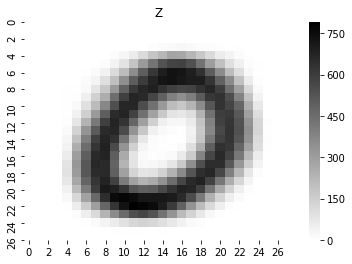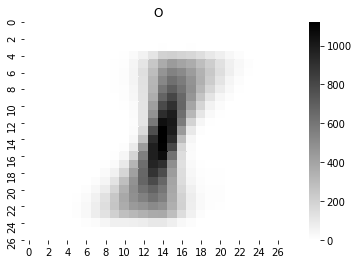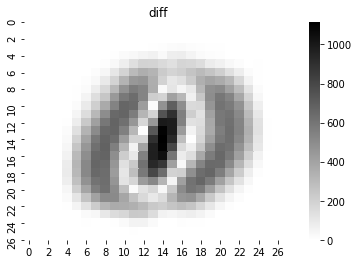The plots we obtain for Z and O are approximately the ones we expect, representing what an average 0 and 1 look like.

diff is also particularly interesting, since it highlights the points that would most help identifying a 0 from a 1. As already mentioned, the center of the grid contains a group of useful pixels. Another area of interest is the circular shape of the zero, which is seldom black for 1's. The whiter areas, instead, are those that are typically either black or white for both digits.

Finally, notice how these considerations on diff only make sense if the classes 0 and 1 have the same number of elements. Otherwise, computing the difference between two umbalanced lists would have resulted in skewed results.

So, let's make sure that our labels have similar numbers of elements.

In :
(labels.count(0), labels.count(1))

Out:
(980, 1135)

The numbers are actually fairly similar, but if we wanted to outdo ourselves, we should have normalized our Z and O before proceeding.

This is how we could do this. The results do not vary significantly, given the already well balanced classes.

In :
Z_count = labels.count(0)
O_count = labels.count(1)
diff_norm = [ abs(z / Z_count - o / O_count) for z,o in zip (Z,O) ]
argmax(diff_norm)

Out:
406